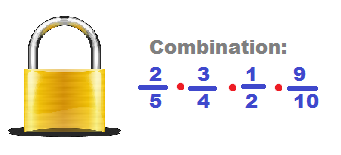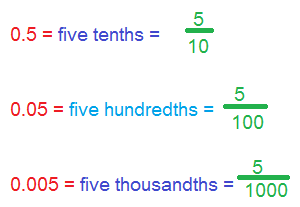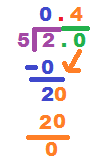# Fractions to Decimals: Lesson for Kids

Instructor: Bethany Calderwood

Bethany has taught special education in grades PK-5 and has a master's degree in special education.

In this lesson, you will learn how fractions and decimals represent parts of a whole. You will learn how to convert fractions into decimals and practice a few examples.

## A Decimal Mystery

Detective Sahmaj is on a quest to find some stolen treasure. His clue is a combination to a locked room inside an abandoned warehouse.When Detective Sahmaj reaches the door, he gets ready to enter the combination into the lock. But to his surprise, the lock can only be opened using numbers in decimal form. But the detective's clue is made of fractions! How will he solve this mystery?

## Parts of a Whole

Fractions and decimals are both ways to represent parts of a whole--both a fraction and a decimal express a number that is less than one. Detective Sahmaj needs to convert his fractions into decimals. Sometimes, in order to complete a math problem, you will also need to convert a fraction into a decimal. Let's look at the process and solve Detective Sahmaj's mystery.

## The Meaning of Decimals

When you read a decimal number, it's easy to see the relationship between decimals and fractions. The decimal number 0.5 is read 'five-tenths.' That is the same way you would read this fraction: 5/10. So, a decimal can be written as a fraction using its place value. Look at these examples:Now that we understand the meaning of decimals, it's time to change fractions into decimals.

## The Meaning of Fractions

The line in a fraction that separates the numerator from the denominator means ''divided by.'' The fraction 2/5 can be read as two fifths, or it can be read as two divided by five. To turn a fraction into a decimal, we divide the numerator by the denominator.

## Converting Fractions to Decimals

Let's convert Detective Sahmaj's first fraction, 2/5, into a decimal. We will review the steps of long division while we solve this problem:To unlock this lesson you must be a Study.com Member.

### Register to view this lesson

Are you a student or a teacher?

#### See for yourself why 30 million people use Study.com

##### Become a Study.com member and start learning now.
Back
What teachers are saying about Study.com

### Earning College Credit

Did you know… We have over 200 college courses that prepare you to earn credit by exam that is accepted by over 1,500 colleges and universities. You can test out of the first two years of college and save thousands off your degree. Anyone can earn credit-by-exam regardless of age or education level.